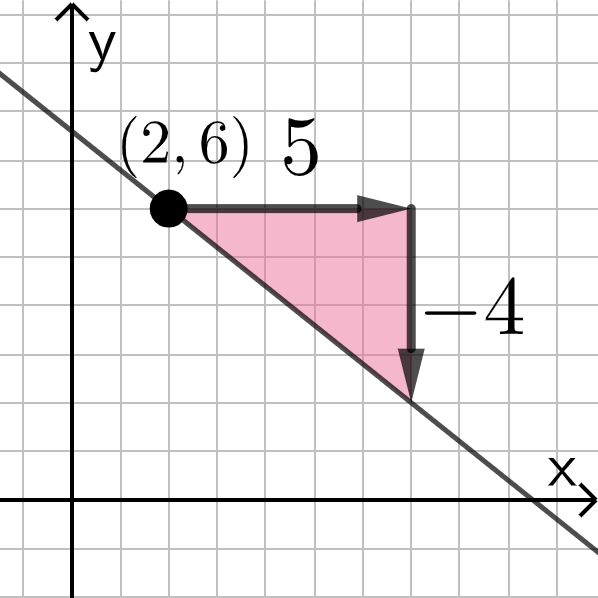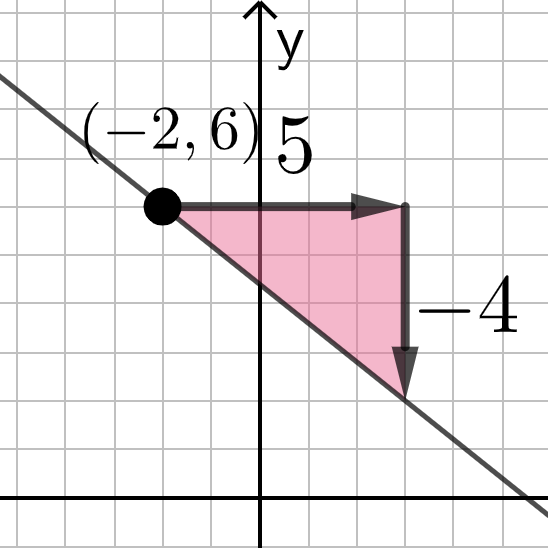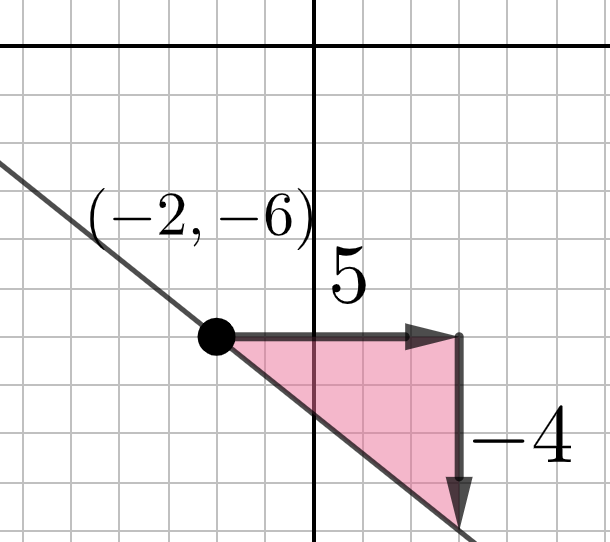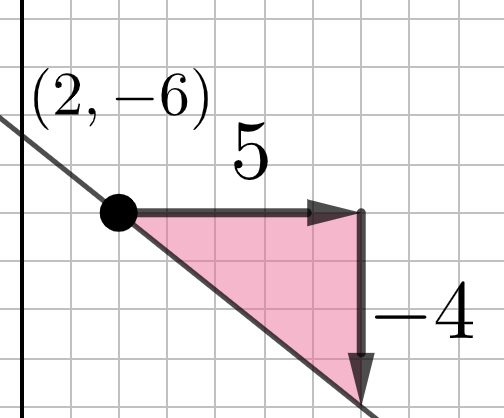Point-Slope Form: Quick Intro

The LARGE POINT (left) and sliders (right) are moveable. Interact with this app for a few minutes. Then answer the questions that follow.

What do you notice here? Be specific!

What is the equation of the line shown here?Check all that apply
• A
• B
• C
• D

What is the equation of the line shown here?Check all that apply
• A
• B
• C
• D

What is the equation of the line shown here?Check all that apply
• A
• B
• C
• D

What is the equation of the line shown here?Check all that apply
• A
• B
• C
• D

Through which point does the line pass through?

Check all that apply
• A
• B
• C
• D